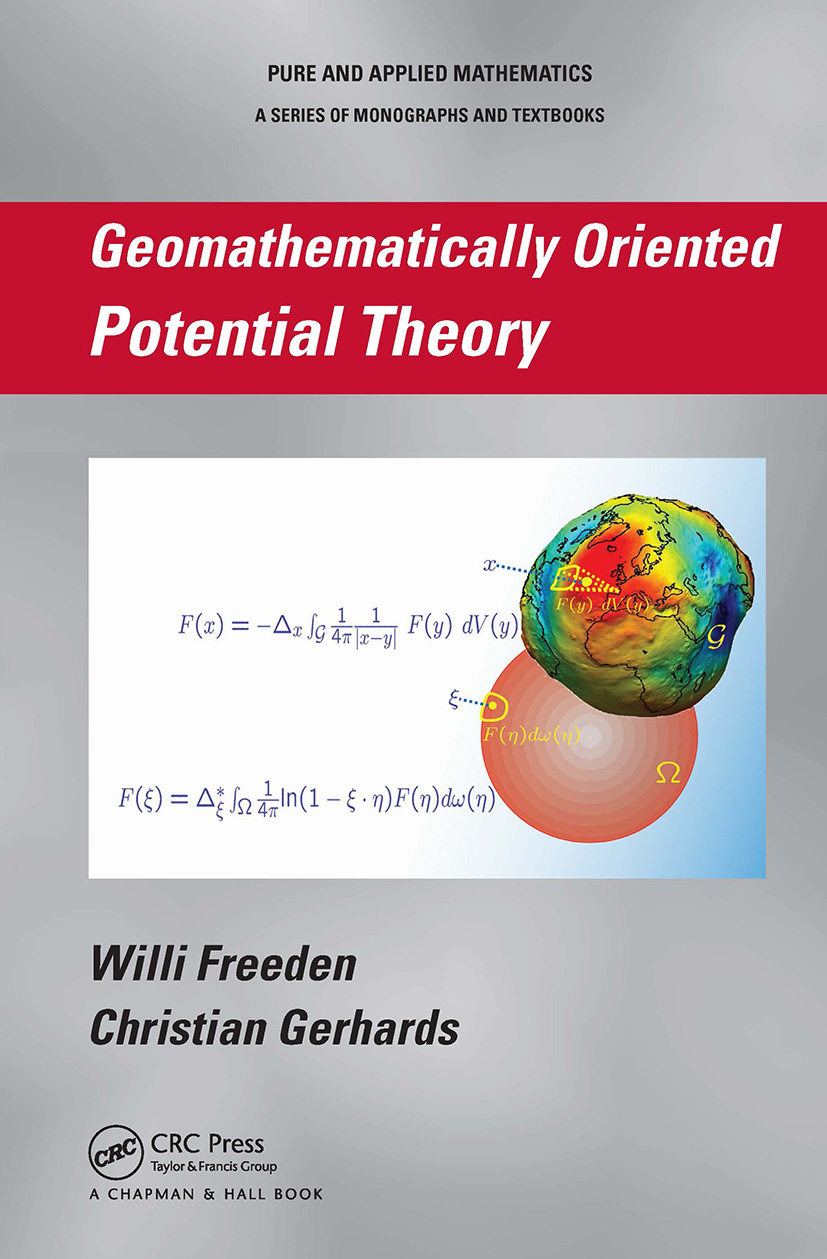# Geomathematically Oriented Potential Theory

## 1st Edition

Chapman and Hall/CRC

468 pages | 57 B/W Illus.

##### Purchasing Options:\$ = USD
Hardback: 9781439895429
pub: 2012-10-30
\$150.00
x
eBook (VitalSource) : 9780429065538
pub: 2012-10-30
from \$28.98

FREE Standard Shipping!

### Description

As the Earth`s surface deviates from its spherical shape by less than 0.4 percent of its radius and today’s satellite missions collect their gravitational and magnetic data on nearly spherical orbits, sphere-oriented mathematical methods and tools play important roles in studying the Earth’s gravitational and magnetic field.

Geomathematically Oriented Potential Theory presents the principles of space and surface potential theory involving Euclidean and spherical concepts. The authors offer new insight on how to mathematically handle gravitation and geomagnetism for the relevant observables and how to solve the resulting potential problems in a systematic, mathematically rigorous framework.

The book begins with notational material and the necessary mathematical background. The authors then build the foundation of potential theory in three-dimensional Euclidean space and its application to gravitation and geomagnetism. They also discuss surface potential theory on the unit sphere along with corresponding applications.

Focusing on the state of the art, this book breaks new geomathematical grounds in gravitation and geomagnetism. It explores modern sphere-oriented potential theoretic methods as well as classical space potential theory.

PRELIMINARIES

Three-Dimensional Euclidean Space R3

Basic Notation

Integral Theorems

Two-Dimensional Sphere Ω

Basic Notation

Integral Theorems

(Scalar) Spherical Harmonics

(Scalar) Circular Harmonics

Vector Spherical Harmonics

Tensor Spherical Harmonics

POTENTIAL THEORY IN THE EUCLIDEAN SPACE R3

Basic Concepts

Background Material

Volume Potentials

Surface Potentials

Boundary-Value Problems

Locally and Globally Uniform Approximation

Gravitation

Oblique Derivative Problem

Satellite Problems

Gravimetry Problem

Geomagnetism

Geomagnetic Background

Mie and Helmholtz Decomposition

Gauss Representation and Uniqueness

Separation of Sources

Ionospheric Current Systems

POTENTIAL THEORY ON THE UNIT SPHERE Ω

Basic Concepts

Background Material

Surface Potentials

Curve Potentials

Boundary-Value Problems

Differential Equations for Surface Gradient and Surface Curl Gradient

Locally and Globally Uniform Approximation

Gravitation

Disturbing Potential

Linear Regularization Method

Multiscale Solution

Geomagnetics

Mie and Helmholtz Decomposition

Higher-Order Regularization Methods

Separation of Sources

Ionospheric Current Systems

Bibliography

Index

Exercises appear at the end of each chapter.

### About the Authors

Willi Freeden is a professor in the Geomathematics Group at the University of Kaiserslautern. Dr. Freeden is an editorial board member of seven international journals and was previously the editor-in-chief of the International Journal on Geomathematics. His research interests include special functions of mathematical (geo)physics, PDEs, constructive approximation, integral transforms, numerical methods, the use of mathematics in industry, and inverse problems in geophysics, geodesy, and satellite technology.

Christian Gerhards is a visiting postdoc researcher in the Department of Mathematics and Statistics at the University of New South Wales.

### Subject Categories

##### BISAC Subject Codes/Headings:
MAT003000
MATHEMATICS / Applied
SCI032000
SCIENCE / Geophysics
TEC010000
TECHNOLOGY & ENGINEERING / Environmental / General